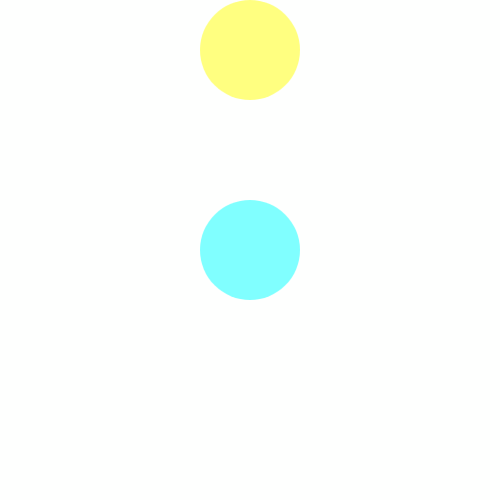# teaching machines

## With Blocks in Twoville

In my software engineering class in college, Dr. Drake dropped on us a half-written piece of software that managed a database of movies. Our challenge was to improve it. It was the first and only time I’ve touched Visual Basic, and I remember only one thing about the project and the language: with-statements.

Suppose you’re rattling off a sequence of references to an object:

hero.Name = "Tenshi"
hero.HitPoints = 100
hero.Mana = 40
hero.ClearEffects()


Notice that every line starts with hero. A with-statement factors out this object prefix:

with hero
.Name = "Tenshi"
.HitPoints = 100
.Mana = 40
.ClearEffects()
end


20-year-old me thought with-statements were fun. Fast-forward to today. 37-year-old me still thinks with-statements are fun.

In Twoville, the programmer creates a shape and then sets many properties to orient and color the shape. There are so many properties to set that I decided to leave them all out of the constructor. For example, here we create a square with a black outline:

square = rectangle()
square.position = [0, 0]
square.size = [100, 100]
square.rgb = [1, 0, 1]
square.stroke.rgb = [0, 0, 0]
square.stroke.size = 1

I soon tired of writing square so many times, so I added with-statements to Twoville. Now I can write this:

square = rectangle()
with square
position = [0, 0]
size = [100, 100]
rgb = [1, 0, 1]
stroke.rgb = [0, 0, 0]
stroke.size = 1

I can even deny the square the privilege of having a name:

with rectangle()
position = [0, 0]
size = [100, 100]
rgb = [1, 0, 1]
stroke.rgb = [0, 0, 0]
stroke.size = 1

During a talk yesterday I sketched out an animation of two circles dancing together forever using with-statements. Both follow a concentric figure-8 pattern, but one travels horizontally and the other vertically. Here’s the end result:And here’s the Twoville code that produced this animation:

with t
start = 0
stop = 40

# Until we get a background command, let's just splat
# a big white rectangle across the canvas.
with rectangle()
position = [0, 0]
size = [1000, 1000]
rgb = [1, 1, 1]

# Horizontal circle
with circle()
opacity = 0.5
rgb = [0, 1, 1]
position = [400, 300]

# The first phase is a loop around the left.
0 -> t -> 20
pivot = [300, 300]
0 -> t
rotation = 0
t -> 20
rotation = 360

# The second phase is a loop around the right.
20 -> t -> 40
pivot = [500, 300]
20 -> t
rotation = 0
t -> 40
rotation = -360

# Vertical circle
with circle()
opacity = 0.5
rgb = [1, 1, 0]
position = [400, 300]

# The first phase is a half-loop around the top-left.
0 -> t -> 10
pivot = [400, 200]
0 -> t
rotation = 180
t -> 10
rotation = 0

# The second phase loops around the bottom.
10 -> t -> 30
pivot = [400, 400]
10 -> t
rotation = 0
t -> 30
rotation = 360

# The third phase is a half-loop around the top-right.
30 -> t -> 40
pivot = [400, 200]
30 -> t
rotation = 0
t -> 40
rotation = -180

I wondered if there was a way to situate and spin the circles so that they never collide. After a few experiments, I’m mostly certain that there will always be a collision somewhere. But I haven’t worked out a formal proof and never will—unless I get bored in a talk.